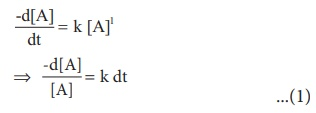Home | | Chemistry 12th Std | The integrated rate equation

# The integrated rate equation

Rate of change of concentration of the reactant is directly proportional to that of concentration of the reactant.

The integrated rate equation:

We have just learnt that the rate of change of concentration of the reactant is directly proportional to that of concentration of the reactant. For a general reaction,

A product

The rate law isWhere k is the rate constant, and x is the order of the reaction. The above equation is a differential equation, -d[A]/dt so it gives the rate at any instant. However, using the above expression, we cannot answer questions such as how long will it take for a specific concentration of A to be used up in the reaction? What will be the concentration of reactant after a time ‘ t ’?. To answer such questions, we need the integrated form of the above rate law which contains time as a variable.

## 1. Integrated rate law for a first order reaction

A reaction whose rate depends on the reactant concentration raised to the first power is called a first order reaction. Let us consider the following Cl2 first order reaction,

A product

Rate law can be expressed as

Rate = k [A]1

Where, k is the first order rate constant.Integrate the above equation between the limits of time t = 0 and time equal to t, while the concentration varies from the initial concentration [A0 ] to [A] at the later time.This equation is in natural logarithm. To convert it into usual logarithm with base 10, we have to multiply the term by 2.303.Equation (2) can be written in the form y = mx + c as below

ln [A0 ] ln [A] = kt

ln [A] = ln [A0 ] kt

y = c + mx

If we follow the reaction by measuring the concentration of the reactants at regular time interval‘t’, a plot of ln[A] against ‘t’ yields a straight line with a negative slope.From this, the rate constant is calculated.

Examples for the first order reaction

(i) Decomposition of dinitrogen pentoxide(ii) Decomposition of thionylchloride; SO2Cl2 (l) →SO2 (g) + Cl2 (g)

(iii) Decomposition of the H2O2  in aqueous solution; H2O2 (aq) → H2O(l) + ½ O2(g)

(iv) Isomerisation of cyclopropane to propene.

### Pseudo first order reaction:

Kinetic study of a higher order reaction is difficult to follow, for example, in a study of a second order reaction involving two different reactants; the simultaneous measurement of change in the concentration of both the reactants is very difficult. To overcome such difficulties, A second order reaction can be altered to a first order reaction by taking one of the reactant in large excess, such reaction is called pseudo first order reaction. Let us consider the acid hydrolysis of an ester,

CH3 COOCH3 (aq) + H2 O (l) H + CH3COOH (aq) + CH3OH (aq)Rate = k [CH3 COOCH3 ] [H2O]

If the reaction is carried out with the large excess of water, there is no significant change in the concentration of water during hydrolysis. i.e.,concentration of water remains almost a constant.

Now, we can define k [H2O] = k' ; Therefore the above rate equation becomes

Rate = k' [CH3COOCH3 ]

Thus it follows first order kinetics.

## 2. Integrated rate law for a zero order reaction:

A reaction in which the rate is independent of the concentration of the reactant over a wide range of concentrations is called as zero order reactions. Such reactions are rare. Let us consider the following hypothetical zero order reaction.

A→productThe rate law can be written as,

Rate = k [A]0Integrate the above equation between the limits of [A0] at zero time and [A] at some later time 't',Equation (2) is in the form of a straight

line y = mx + c

Ie., [A] = −kt + [A0 ]

y = c + mx

A plot of [A] Vs time gives a straight line with a slope of k and y - intercept of [A0 ] .

### Examples for a zero order reaction:

1. Photochemical reaction between H2 and I2

H2 (g)+Cl2 (g) hv2HCl(g)2. Decomposition of N2O on hot platinum surface3. Iodination of acetone in acid medium is zero order with respect to iodine.

CH3COCH3 + I2 H ICH2COCH3 + HIRate = k [CH3COCH3 ] [H+ ]Tags : Chemical Kinetics , 12th Chemistry : UNIT 7 : Chemical Kinetics
Study Material, Lecturing Notes, Assignment, Reference, Wiki description explanation, brief detail
12th Chemistry : UNIT 7 : Chemical Kinetics : The integrated rate equation | Chemical Kinetics